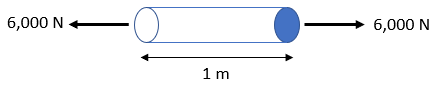$$E=\frac{\sigma}{\varepsilon}=\frac{P/A}{\delta/L}$$$where $$P$$ is loading, $$L$$ is the length of member, $$A$$ is the cross sectional area, $$\delta$$ is the deformation, $$\sigma$$ is the stress, $$\varepsilon$$ is the engineering strain, and $$E$$ is the modulus of elasticity Hint 2 For uniaxial deformation: $$\delta =\frac{PL}{AE}$$$
where $$P$$ is loading, $$L$$ is the length of member, $$A$$ is the cross sectional area, and $$E$$ is the modulus of elasticity
$$Deformation=\delta =\frac{PL}{AE}$$$where $$P$$ is loading, $$L$$ is the length of member, $$A$$ is the cross sectional area, and $$E$$ is the modulus of elasticity Thus, $$\delta =\frac{(6,000N)(1m)}{(2,000\times 10^{-6}m^2)(200\times 10^9\frac{N}{m^2})}$$$
$$=\frac{6000}{400,000,000}m=0.000015m=15\: \mu m$$$$$15\: \mu m$$$